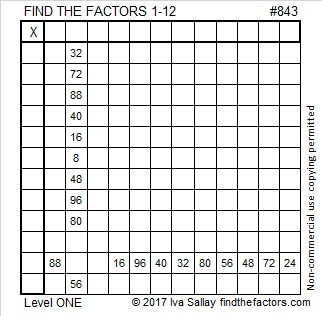# 843 is a Lucas NumberPrint the puzzles or type the solution on this excel file: 12 factors 843-852

843 is the hypotenuse of Pythagorean triple 480-693-843 which is 3 times (160-231-281).

I think 843 looks interesting in a couple different bases:

• It is palindrome 1101001011 in BASE 2 because 2⁹ + 2⁸ + 2⁶ + 2³ + 2¹ + 2⁰ = 843
• It is 123 in BASE 28 because 1(28²) + 2(28¹) + 3(28º) = 843

From Stetson.edu, I learned that 843 is the 14th Lucas number which is similar to being a Fibonacci number.

• Fibonacci numbers start with 1, 1, and all the rest of the Fibonacci numbers are found by adding the previous two numbers in the series.
• Lucas numbers start with 2, 1, and all the rest of the Lucas numbers are found by adding the previous two numbers in the series.

843 makes the list of Lucas numbers: 2, 1, 3, 4, 7, 11, 18, 29, 47, 76, 123, 199, 322, 521, 843, . . .

Even though it is the 15th number on the list, it is the 14th Lucas number. (The list starts with the zeroth number?)

Here is 843’s factoring information:

• 843 is a composite number.
• Prime factorization: 843 = 3 × 281
• The exponents in the prime factorization are 1 and 1. Adding one to each and multiplying we get (1 + 1)(1 + 1) = 2 × 2 = 4. Therefore 843 has exactly 4 factors.
• Factors of 843: 1, 3, 281, 843
• Factor pairs: 843 = 1 × 843 or 3 × 281
• 843 has no square factors that allow its square root to be simplified. √843 ≈ 03446228